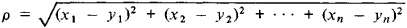# Multidimensional Space

The following article is from The Great Soviet Encyclopedia (1979). It might be outdated or ideologically biased.

## Multidimensional Space

a space having more than three dimensions. Ordinary Euclidean space studied in elementary geometry is three dimensional, planes are two dimensional, and lines are one dimensional. The concept of a multidimensional space arose in the process of the generalization of the subject of geometry. The discovery of relations and forms for many classes of mathematical objects (some lacking any geometric interpretation) that are similar to the relations and forms of three-dimensional space lay at the basis of this process. In the course of this process, there was a gradual crystallization of the concept of an abstract mathematical space as a system of elements of any type between which there exist relations that are similar to various important relations between points of ordinary space. This concept found its most general expression in such concepts as topological space and, in particular, metric space.

The simplest multidimensional spaces are n-dimensional Euclidean spaces, where n can be any natural number. A “point” in n-dimensional Euclidean space is specified by the n “coordinates” x1, X2, … , xn, which may assume any real values, in a manner similar to the manner in which the position of a point in ordinary Euclidean space is defined by specifying the three rectangular coordinates of the point. The distance p between the two points M(x1, x2, …, xn and M’(y1, y2, … ,yn) is defined by the formulawhich is similar to the formula for the distance between two points in ordinary Euclidean space. Other geometric concepts can also be generalized to the case of n-dimensional space by preserving this analogy. Thus, in multidimensional spaces, we consider not only two-dimensional planes but also k-dimensional planes (k < n), which, as in ordinary Euclidean space, are defined by linear equations or by systems of such equations.

The concept of an n-dimensional Euclidean space has important applications in the theory of functions of several variables, permitting a function of n variables to be treated as a function of a point of this space. Thus, geometrical representations and methods can also be used for studying functions of any number of variables (not only for one, two, or three variables). This was the chief stimulus to the formulation of the concept of an n-dimensional Euclidean space.

Other multidimensional spaces also play important roles. Thus, the four-dimensional space whose elements are “world-points” is used in discussing the physical principle of relativity. In the concept of a world-point (unlike a point in ordinary space), a fixed position in space is connected with a fixed position in time; thus, world-points are specified by four coordinates rather than three. It is natural to take the expression.

(M’M”)2 = (x’ − x”)2 + (y’ − y”)2 + (z’ − z”)2c2(t’ − t”)2

in which c is the velocity of light, as the square of the “distance” between the world-points M’(x’, y’, z’, t’) and M”(x”, y”, z”, t”), where the first three “coordinates” are space coordinates and the fourth is the time coordinate. This space is “pseudo-Euclidean,” since the last term of the equation is negative.

In general, a topological space that has dimension n at each point is said to be an n-dimensional space. In the most important cases, this means that every point has a neighborhood that is homeomorphic to an open sphere of n-dimensional Euclidean space. (See GEOMETRY for additional details on the development of the concept of a multidimensional space and for references.)

The Great Soviet Encyclopedia, 3rd Edition (1970-1979). © 2010 The Gale Group, Inc. All rights reserved.
References in periodicals archive ?
It plotted the different images in a multidimensional space, with more similar butterflies closer together and less similar butterflies further apart.
The volume begins powerfully, imagining a U.S.-Mexico border that morphs into a metaphorical, multidimensional space. Other stories call forth equally engaging situations: A disapproving father reunites with a son who has been modified with gills to live underwater.
Create a multidimensional space. "We want to give students a choice and agency for using the space, so they can reshape it for their needs," he said.
In the current study, Tsao and postdoctoral fellow Steven Le Chang, the paper's first author, found that rather than representing a specific identity, each face cell represents a specific axis within a multidimensional space, which they call the face space.
A machine learning method that calculates hyperplanes in multidimensional space, and that can accurately separate data.
Composed in a dazzling formal idiom that serves its scholarly function, this book invites readers to wander and wonder amid the eco-fantasies enacted in the multidimensional space of the first public theaters.
where C is the correlation dimension; r is the radius of the region in multidimensional space.
Rakotoson  studied a generalized thin-film equation with period boundary in multidimensional space. Moreover, the paper  investigated the existence of the weak solutions and strong solutions with the initial function near a positive constant steady state solution.
Correspondence analysis essentially maps counts from cross tabulations in multidimensional space. Unlike network analysis, used by many researchers who study social movements to increasingly understand discursive successes of framing mobilization (e.g.
Behind the HBMP technology is a new mathematical algorithm that maps the compressed multidimensional space in-memory, through bit-level structures.
Farassat, The integration of [delta]'(f) in a multidimensional space, J.

Site: Follow: Share:
Open / Close